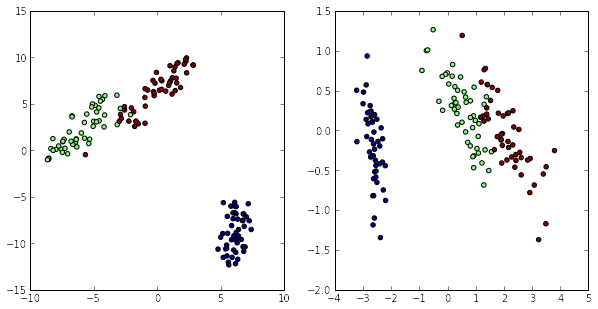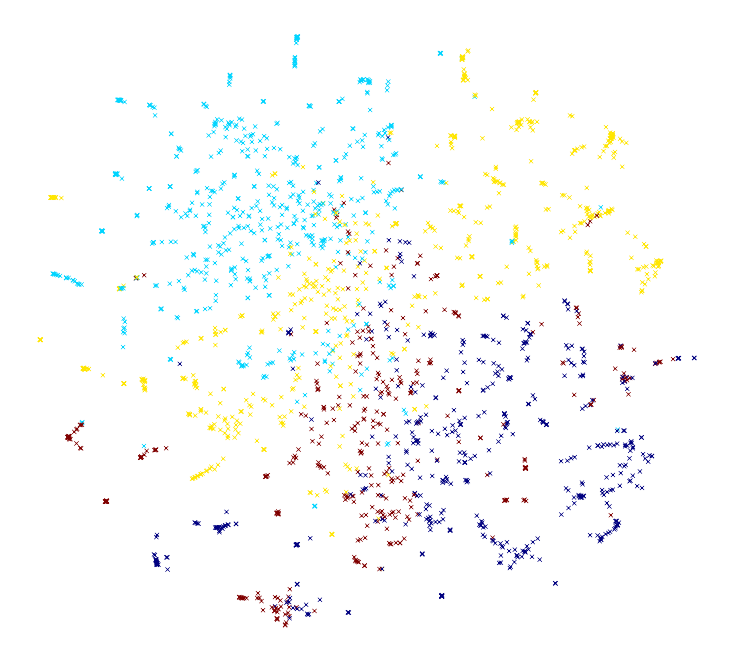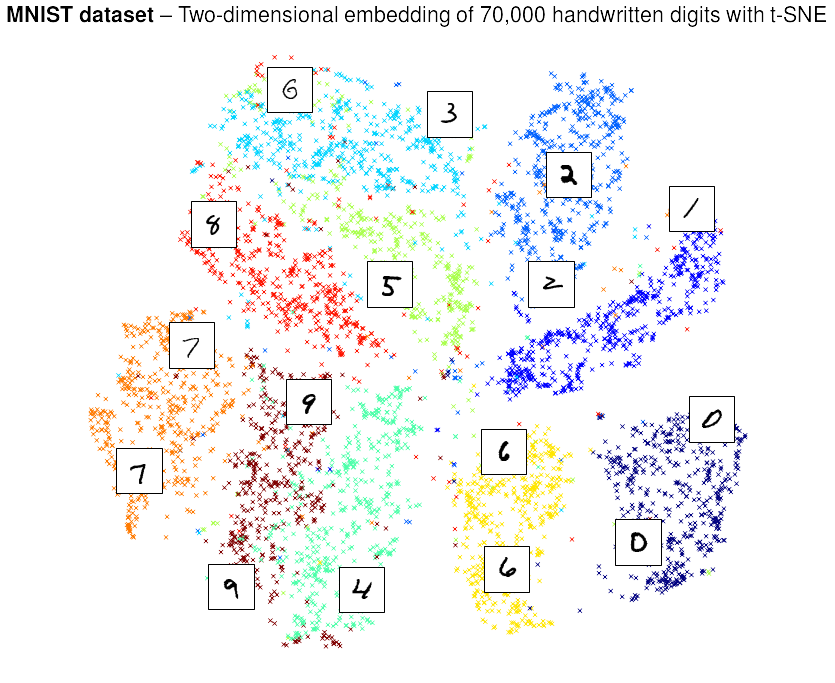In :
%pylab inline

Populating the interactive namespace from numpy and matplotlib


# t-Distributed Stochastic Neighbor Embedding (t-SNE) in sklearn¶

t-SNE is a tool for data visualization. It reduces the dimensionality of data to 2 or 3 dimensions so that it can be plotted easily. Local similarities are preserved by this embedding.

t-SNE converts distances between data in the original space to probabilities. First, we compute conditional probabilites

$$p_{j|i} = \frac{\exp{(-d(\boldsymbol{x}_i, \boldsymbol{x}_j) / (2 \sigma_i^2)})}{\sum_{i \neq k} \exp{(-d(\boldsymbol{x}_i, \boldsymbol{x}_k) / (2 \sigma_i^2)})}, \quad p_{i|i} = 0,$$

which will be used to generate joint probabilities

$$p_{ij} = \frac{p_{j|i} + p_{i|j}}{2N}.$$

The $\sigma_i$ will be determined automatically. This procedure can be influenced by setting the perplexity of the algorithm.

A heavy-tailed distribution will be used to measure the similarities in the embedded space

$$q_{ij} = \frac{(1 + ||\boldsymbol{y}_i - \boldsymbol{y}_j)||^2)^{-1}}{\sum_{k \neq l} (1 + ||\boldsymbol{y}_k - \boldsymbol{y}_l)||^2)^{-1}},$$

and then we minimize the Kullback-Leibler divergence

$$KL(P|Q) = \sum_{i \neq j} p_{ij} \log \frac{p_{ij}}{q_{ij}}$$

between both distributions with gradient descent (and some tricks). Note that the cost function is not convex and multiple runs might yield different results.

More information can be found in these resources and in the documentation from t-SNE:

In :
from sklearn.manifold import TSNE

help(TSNE)

Help on class TSNE in module sklearn.manifold.t_sne:

class TSNE(sklearn.base.BaseEstimator)
|  t-distributed Stochastic Neighbor Embedding.
|
|  t-SNE  is a tool to visualize high-dimensional data. It converts
|  similarities between data points to joint probabilities and tries
|  to minimize the Kullback-Leibler divergence between the joint
|  probabilities of the low-dimensional embedding and the
|  high-dimensional data. t-SNE has a cost function that is not convex,
|  i.e. with different initializations we can get different results.
|
|  It is highly recommended to use another dimensionality reduction
|  method (e.g. PCA for dense data or TruncatedSVD for sparse data)
|  to reduce the number of dimensions to a reasonable amount (e.g. 50)
|  if the number of features is very high. This will suppress some
|  noise and speed up the computation of pairwise distances between
|  samples. For more tips see Laurens van der Maaten's FAQ .
|
|  Parameters
|  ----------
|  n_components : int, optional (default: 2)
|      Dimension of the embedded space.
|
|  perplexity : float, optional (default: 30)
|      The perplexity is related to the number of nearest neighbors that
|      is used in other manifold learning algorithms. Larger datasets
|      usually require a larger perplexity. Consider selcting a value
|      between 5 and 50. The choice is not extremely critical since t-SNE
|      is quite insensitive to this parameter.
|
|  early_exaggeration : float, optional (default: 4.0)
|      Controls how tight natural clusters in the original space are in
|      the embedded space and how much space will be between them. For
|      larger values, the space between natural clusters will be larger
|      in the embedded space. Again, the choice of this parameter is not
|      very critical. If the cost function increases during initial
|      optimization, the early exaggeration factor or the learning rate
|      might be too high.
|
|  learning_rate : float, optional (default: 1000)
|      The learning rate can be a critical parameter. It should be
|      between 100 and 1000. If the cost function increases during initial
|      optimization, the early exaggeration factor or the learning rate
|      might be too high. If the cost function gets stuck in a bad local
|      minimum increasing the learning rate helps sometimes.
|
|  n_iter : int, optional (default: 1000)
|      Maximum number of iterations for the optimization. Should be at
|      least 200.
|
|  metric : string or callable, (default: "euclidean")
|      The metric to use when calculating distance between instances in a
|      feature array. If metric is a string, it must be one of the options
|      allowed by scipy.spatial.distance.pdist for its metric parameter, or
|      a metric listed in pairwise.PAIRWISE_DISTANCE_FUNCTIONS.
|      If metric is "precomputed", X is assumed to be a distance matrix.
|      Alternatively, if metric is a callable function, it is called on each
|      pair of instances (rows) and the resulting value recorded. The callable
|      should take two arrays from X as input and return a value indicating
|      the distance between them.
|
|  init : string, optional (default: "random")
|      Initialization of embedding. Possible options are 'random' and 'pca'.
|      PCA initialization cannot be used with precomputed distances and is
|      usually more globally stable than random initialization.
|
|  verbose : int, optional (default: 0)
|      Verbosity level.
|
|  random_state : int or RandomState instance or None (default)
|      Pseudo Random Number generator seed control. If None, use the
|      numpy.random singleton. Note that different initializations
|      might result in different local minima of the cost function.
|
|  Attributes
|  ----------
|  embedding_ : array-like, shape (n_samples, n_components)
|      Stores the embedding vectors.
|
|  training_data_ : array-like, shape (n_samples, n_features)
|      Stores the training data.
|
|  Examples
|  --------
|
|  >>> import numpy as np
|  >>> from sklearn.manifold import TSNE
|  >>> X = np.array([[0, 0, 0], [0, 1, 1], [1, 0, 1], [1, 1, 1]])
|  >>> model = TSNE(n_components=2, random_state=0)
|  >>> model.fit_transform(X) # doctest: +ELLIPSIS, +NORMALIZE_WHITESPACE
|  array([[  887.28...,   238.61...],
|         [ -714.79...,  3243.34...],
|         [  957.30..., -2505.78...],
|         [-1130.28...,  -974.78...])
|
|  References
|  ----------
|
|   van der Maaten, L.J.P.; Hinton, G.E. Visualizing High-Dimensional Data
|      Using t-SNE. Journal of Machine Learning Research 9:2579-2605, 2008.
|
|   van der Maaten, L.J.P. t-Distributed Stochastic Neighbor Embedding
|      http://homepage.tudelft.nl/19j49/t-SNE.html
|
|  Method resolution order:
|      TSNE
|      sklearn.base.BaseEstimator
|      __builtin__.object
|
|  Methods defined here:
|
|  __init__(self, n_components=2, perplexity=30.0, early_exaggeration=4.0, learning_rate=1000.0, n_iter=1000, metric='euclidean', init='random', verbose=0, random_state=None)
|
|  fit_transform(self, X)
|      Transform X to the embedded space.
|
|      Parameters
|      ----------
|      X : array, shape (n_samples, n_features) or (n_samples, n_samples)
|          If the metric is 'precomputed' X must be a square distance
|          matrix. Otherwise it contains a sample per row.
|
|      Returns
|      -------
|      X_new : array, shape (n_samples, n_components)
|          Embedding of the training data in low-dimensional space.
|
|  ----------------------------------------------------------------------
|  Methods inherited from sklearn.base.BaseEstimator:
|
|  __repr__(self)
|
|  get_params(self, deep=True)
|      Get parameters for this estimator.
|
|      Parameters
|      ----------
|      deep: boolean, optional
|          If True, will return the parameters for this estimator and
|          contained subobjects that are estimators.
|
|      Returns
|      -------
|      params : mapping of string to any
|          Parameter names mapped to their values.
|
|  set_params(self, **params)
|      Set the parameters of this estimator.
|
|      The method works on simple estimators as well as on nested objects
|      (such as pipelines). The former have parameters of the form
|      <component>__<parameter> so that it's possible to update each
|      component of a nested object.
|
|      Returns
|      -------
|      self
|
|  ----------------------------------------------------------------------
|  Data descriptors inherited from sklearn.base.BaseEstimator:
|
|  __dict__
|      dictionary for instance variables (if defined)
|
|  __weakref__
|      list of weak references to the object (if defined)



## A simple example: the Iris dataset¶

In :
from sklearn.datasets import load_iris
from sklearn.decomposition import PCA

X_tsne = TSNE(learning_rate=100).fit_transform(iris.data)
X_pca = PCA().fit_transform(iris.data)


t-SNE can help us to decide whether classes are separable in some linear or nonlinear representation. Here we can see that the 3 classes of the Iris dataset can be separated quite easily. They can even be separated linearly which we can conclude from the low-dimensional embedding of the PCA.

In :
figure(figsize=(10, 5))
subplot(121)
scatter(X_tsne[:, 0], X_tsne[:, 1], c=iris.target)
subplot(122)
scatter(X_pca[:, 0], X_pca[:, 1], c=iris.target)

Out:
<matplotlib.collections.PathCollection at 0x4a9ea90>## High-dimensional sparse data: the 20 newsgroups dataset¶

In high-dimensional and nonlinear domains, PCA is not applicable any more and many other manifold learning algorithms do not yield good visualizations either because they try to preserve the global data structure.

In :
from sklearn.datasets import fetch_20newsgroups
from sklearn.feature_extraction.text import TfidfVectorizer

categories = ['alt.atheism', 'talk.religion.misc', 'comp.graphics', 'sci.space']
newsgroups = fetch_20newsgroups(subset="train", categories=categories)
vectors = TfidfVectorizer().fit_transform(newsgroups.data)

In :
print(repr(vectors))

<2034x34118 sparse matrix of type '<type 'numpy.float64'>'
with 323433 stored elements in Compressed Sparse Row format>


For high-dimensional sparse data it is helpful to first reduce the dimensions to 50 dimensions with TruncatedSVD and then perform t-SNE. This will usually improve the visualization.

In :
from sklearn.decomposition import TruncatedSVD

X_reduced = TruncatedSVD(n_components=50, random_state=0).fit_transform(vectors)

In :
X_embedded = TSNE(n_components=2, perplexity=40, verbose=2).fit_transform(X_reduced)

[t-SNE] Computing pairwise distances...
[t-SNE] Computed conditional probabilities for sample 1000 / 2034
[t-SNE] Computed conditional probabilities for sample 2000 / 2034
[t-SNE] Computed conditional probabilities for sample 2034 / 2034
[t-SNE] Mean sigma: 0.102335
[t-SNE] Iteration 10: error = 22.2322467, gradient norm = 0.0800006
[t-SNE] Iteration 20: error = 20.1076571, gradient norm = 0.0764023
[t-SNE] Iteration 30: error = 19.6108777, gradient norm = 0.0738040
[t-SNE] Iteration 40: error = 19.3559024, gradient norm = 0.0719897
[t-SNE] Iteration 50: error = 19.4768306, gradient norm = 0.0696084
[t-SNE] Iteration 60: error = 18.6167100, gradient norm = 0.0793421
[t-SNE] Iteration 70: error = 19.1011108, gradient norm = 0.0746908
[t-SNE] Iteration 80: error = 18.9686801, gradient norm = 0.0710730
[t-SNE] Iteration 83: did not make any progress during the last 30 episodes. Finished.
[t-SNE] Error after 83 iterations with early exaggeration: 18.940860
[t-SNE] Iteration 90: error = 2.1820063, gradient norm = 0.0156060
[t-SNE] Iteration 100: error = 1.5909306, gradient norm = 0.0078247
[t-SNE] Iteration 110: error = 1.4329588, gradient norm = 0.0032823
[t-SNE] Iteration 120: error = 1.3741529, gradient norm = 0.0017929
[t-SNE] Iteration 130: error = 1.3469589, gradient norm = 0.0022025
[t-SNE] Iteration 140: error = 1.3327666, gradient norm = 0.0026223
[t-SNE] Iteration 150: error = 1.3257519, gradient norm = 0.0031263
[t-SNE] Iteration 160: error = 1.3242508, gradient norm = 0.0036040
[t-SNE] Iteration 170: error = 1.3235266, gradient norm = 0.0039417
[t-SNE] Iteration 180: error = 1.3263037, gradient norm = 0.0041935
[t-SNE] Iteration 190: error = 1.3278295, gradient norm = 0.0044735
[t-SNE] Iteration 195: did not make any progress during the last 30 episodes. Finished.
[t-SNE] Error after 195 iterations: 1.336772

In :
fig = figure(figsize=(10, 10))
ax = axes(frameon=False)
setp(ax, xticks=(), yticks=())
wspace=0.0, hspace=0.0)
scatter(X_embedded[:, 0], X_embedded[:, 1],
c=newsgroups.target, marker="x")

Out:
<matplotlib.collections.PathCollection at 0x81d72d0># MNIST dataset¶

In :
from sklearn.datasets import fetch_mldata

mnist = fetch_mldata("MNIST original")
X, y = mnist.data / 255.0, mnist.target

# Create subset and reduce to first 50 dimensions
indices = arange(X.shape)
random.shuffle(indices)
n_train_samples = 5000
X_pca = PCA(n_components=50).fit_transform(X)
X_train = X_pca[indices[:n_train_samples]]
y_train = y[indices[:n_train_samples]]

In :
# Plotting function
matplotlib.rc('font', **{'family' : 'sans-serif',
'weight' : 'bold',
'size'   : 18})
matplotlib.rc('text', **{'usetex' : True})

def plot_mnist(X, y, X_embedded, name, min_dist=10.0):
fig = figure(figsize=(10, 10))
ax = axes(frameon=False)
title("\\textbf{MNIST dataset} -- Two-dimensional "
"embedding of 70,000 handwritten digits with %s" % name)
setp(ax, xticks=(), yticks=())
wspace=0.0, hspace=0.0)
scatter(X_embedded[:, 0], X_embedded[:, 1],
c=y, marker="x")

if min_dist is not None:
from matplotlib import offsetbox
shown_images = np.array([[15., 15.]])
indices = arange(X_embedded.shape)
random.shuffle(indices)
for i in indices[:5000]:
dist = np.sum((X_embedded[i] - shown_images) ** 2, 1)
if np.min(dist) < min_dist:
continue
shown_images = np.r_[shown_images, [X_embedded[i]]]
imagebox = offsetbox.AnnotationBbox(
offsetbox.OffsetImage(X[i].reshape(28, 28),
cmap=cm.gray_r), X_embedded[i])

In :
X_train_embedded = TSNE(n_components=2, perplexity=40, verbose=2).fit_transform(X_train)

[t-SNE] Computing pairwise distances...
[t-SNE] Computed conditional probabilities for sample 1000 / 5000
[t-SNE] Computed conditional probabilities for sample 2000 / 5000
[t-SNE] Computed conditional probabilities for sample 3000 / 5000
[t-SNE] Computed conditional probabilities for sample 4000 / 5000
[t-SNE] Computed conditional probabilities for sample 5000 / 5000
[t-SNE] Mean sigma: 1.970714
[t-SNE] Iteration 10: error = 24.7854118, gradient norm = 0.0600851
[t-SNE] Iteration 20: error = 23.5140576, gradient norm = 0.0711481
[t-SNE] Iteration 30: error = 21.1764054, gradient norm = 0.0637004
[t-SNE] Iteration 40: error = 20.6641474, gradient norm = 0.0583098
[t-SNE] Iteration 50: error = 20.4368287, gradient norm = 0.0549337
[t-SNE] Iteration 60: error = 17.6146470, gradient norm = 0.0541532
[t-SNE] Iteration 70: error = 17.9446755, gradient norm = 0.0620773
[t-SNE] Iteration 80: error = 18.3607226, gradient norm = 0.0645168
[t-SNE] Iteration 89: did not make any progress during the last 30 episodes. Finished.
[t-SNE] Error after 89 iterations with early exaggeration: 18.662145
[t-SNE] Iteration 90: error = 3.2792420, gradient norm = 0.0142537
[t-SNE] Iteration 100: error = 2.4559145, gradient norm = 0.0062523
[t-SNE] Iteration 110: error = 2.2672422, gradient norm = 0.0027348
[t-SNE] Iteration 120: error = 2.1872763, gradient norm = 0.0017248
[t-SNE] Iteration 130: error = 2.1473784, gradient norm = 0.0015520
[t-SNE] Iteration 140: error = 2.1256136, gradient norm = 0.0014426
[t-SNE] Iteration 150: error = 2.1138911, gradient norm = 0.0014110
[t-SNE] Iteration 160: error = 2.1071622, gradient norm = 0.0013919
[t-SNE] Iteration 170: error = 2.1032282, gradient norm = 0.0013815
[t-SNE] Iteration 180: error = 2.1009067, gradient norm = 0.0013754
[t-SNE] Iteration 190: error = 2.0995291, gradient norm = 0.0013717
[t-SNE] Iteration 200: error = 2.0987087, gradient norm = 0.0013695
[t-SNE] Iteration 210: error = 2.0982191, gradient norm = 0.0013682
[t-SNE] Iteration 220: error = 2.0979266, gradient norm = 0.0013674
[t-SNE] Iteration 230: error = 2.0977516, gradient norm = 0.0013669
[t-SNE] Iteration 240: error = 2.0976469, gradient norm = 0.0013666
[t-SNE] Iteration 250: error = 2.0975843, gradient norm = 0.0013664
[t-SNE] Iteration 260: error = 2.0975468, gradient norm = 0.0013663
[t-SNE] Iteration 270: error = 2.0975243, gradient norm = 0.0013663
[t-SNE] Iteration 280: error = 2.0975109, gradient norm = 0.0013662
[t-SNE] Iteration 290: error = 2.0975028, gradient norm = 0.0013662
[t-SNE] Iteration 300: error = 2.0974980, gradient norm = 0.0013662
[t-SNE] Iteration 310: error = 2.0974951, gradient norm = 0.0013662
[t-SNE] Iteration 320: error = 2.0974934, gradient norm = 0.0013662
[t-SNE] Iteration 326: error difference 0.000000. Finished.
[t-SNE] Error after 326 iterations: 2.097493

In :
plot_mnist(X[indices[:n_train_samples]], y_train, X_train_embedded,
"t-SNE", min_dist=20.0)# Outlook¶

There are some modifications of t-SNE that already have been published. A huge disadvantage of t-SNE is that it scales quadratically with the number of samples ($O(N^2)$) and the optimization is quite slow. These issues and more have been adressed in the following papers: